# 2 Filter过滤法## 2.1 方差过滤

Variance Threshold是通过特征本身方差来筛选特征的类。比如一个特征本身的方差很小，就表示样本在这个特征上基本没有差异，可能特征中的大多数值都一样，甚至整个特征的取值都相同，那这个特征对于样本区分没有什么作用。所以无论接下来的特征工程要做什么，都要优先消除方差为0的特征。VarianceThreshold有重要参数threshold，表示方差的阈值，表示舍弃所有方差小于threshold的特征，不填默认为0，即删除所有的记录都相同的特征。下面代码简单的实现了方差过滤：

```import pandas as pd
from sklearn.feature_selection import VarianceThreshold

x = data.iloc[:,1:]
y = data.iloc[:,0]
print(x.shape)
selector = VarianceThreshold()
x_var0 = selector.fit_transform(x)
print(x_var0.shape)```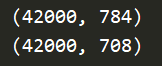```import pandas as pd
import numpy as np
from sklearn.feature_selection import VarianceThreshold

x = data.iloc[:,1:]
y = data.iloc[:,0]
print(x.shape)
selector = VarianceThreshold(np.median(x.var().values))
x_feature_selection = selector.fit_transform(x)
print(x_feature_selection.shape)```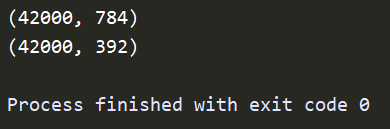```import pandas as pd
import numpy as np
from sklearn.feature_selection import VarianceThreshold
x = data.iloc[:,1:]
y = data.iloc[:,0]
print(x.shape)
selector = VarianceThreshold(0.8 *(1-0.8))
x_feature_selection = selector.fit_transform(x)
print(x_feature_selection.shape)```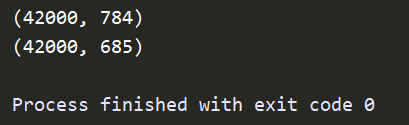K-近邻算法（KNN）是一种比较简单的分类算法，其原理是利用每个样本到其他样本点的距离来判断每个样本点的相似度，然后对样本进行分类。KNN必须遍历每个特征和样本，因而特征越多，KNN所需要的计算力也就越大。

## 2.2 相关性过滤

### 卡方过滤

```from sklearn.ensemble import RandomForestClassifier as RFC
from sklearn.model_selection import cross_val_score
from sklearn.feature_selection import SelectKBest
from sklearn.feature_selection import chi2

#留下300个特征
X_fschi = SelectKBest(chi2, k=300).fit_transform(X_fsvar, y)
X_fschi.shape
#验证模型效果
cross_val_score(RFC(n_estimators=10,random_state=0),X_fschi,y,cv=5).mean()```

K值变化时，模型的评分也会跟着变化，手动去调整一个一个参数的话效率非常低，我们可以使用学习曲线来获得一个最优的超参数K。代码如下：

```import pandas as pd
import numpy as np
from sklearn.feature_selection import VarianceThreshold
from sklearn.ensemble import RandomForestClassifier as RFC
from sklearn.model_selection import cross_val_score
from sklearn.feature_selection import SelectKBest
from sklearn.feature_selection import chi2
import matplotlib.pyplot as plt
x = data.iloc[:,1:]
y = data.iloc[:,0]
# print(x.shape)
selector = VarianceThreshold(np.median(x.var().values))
x_fsvar = selector.fit_transform(x)
score = []
for i in range(390,200,-10):
x_fschi = SelectKBest(chi2,k = i).fit_transform(x_fsvar,y)
once = cross_val_score(RFC(n_estimators=10,random_state=0),x_fschi,y,cv=5).mean()
score.append(once)
plt.plot(range(390,200,-10),score)
plt.show()```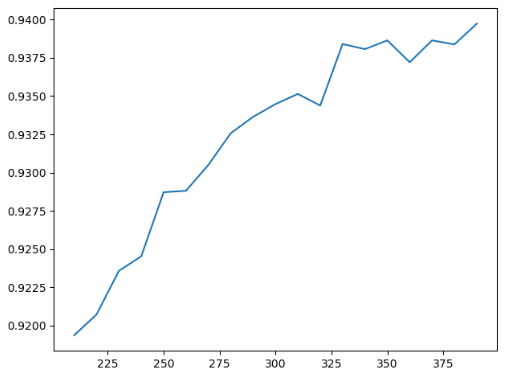p值 <=0.05 或0.01 >0.05 或 0.01 数据差异 差异不是自然形成的 这些差异是很自然的样本误差 相关性 两组数据是相关的 两组数据是相互独立的 原假设 拒绝原假设，接受备择假设 接受原假设

```chivalue, pvalues_chi = chi2(X_fsvar,y)
print(chivalue)
print(pvalues_chi)

#k取多少？我们想要消除所有p值大于设定值，比如0.05或0.01的特征：
k = chivalue.shape - (pvalues_chi > 0.05).sum()

#X_fschi = SelectKBest(chi2, k=填写具体的k).fit_transform(X_fsvar, y)
#cross_val_score(RFC(n_estimators=10,random_state=0),X_fschi,y,cv=5).mean()```

### F检验

F检验，又称ANOVA，方差齐性检验，是用来捕捉每个特征与标签之间的线性关系的过滤方法。它即可以做回归也可以做分类，因此包含feature_selection.f_classif（F检验分类）和feature_selection.f_regression（F检验回归）两个类。其中F检验分类用于标签是离散型变量的数据，而F检验回归用于标签是连续型变量的数据。

F检验的本质是寻找两组数据之间的线性关系，其原假设是”数据不存在显著的线性关系“。它返回F值和p值两个统计量。和卡方过滤一样，我们希望选取p值小于 0.05 或 0.01 的特征，这些特征与标签时显著线性相关的，而p值大于0.05或0.01的特征则被我们认为是和标签没有显著线性关系的特征，应该被删除。以F检验的分类为例，我们继续在数字数据集上来进行特征选择：

```chivalue, pvalues_chi = chi2(X_fsvar,y)
chivalue
pvalues_chi
#k取多少？我们想要消除所有p值大于设定值，比如0.05或0.01的特征：
k = chivalue.shape - (pvalues_chi > 0.05).sum()
#X_fschi = SelectKBest(chi2, k=填写具体的k).fit_transform(X_fsvar, y)
#cross_val_score(RFC(n_estimators=10,random_state=0),X_fschi,y,cv=5).mean()
from sklearn.feature_selection import f_classif
F, pvalues_f = f_classif(X_fsvar,y)
F
pvalues_f
k = F.shape - (pvalues_f > 0.05).sum()
#X_fsF = SelectKBest(f_classif, k=填写具体的k).fit_transform(X_fsvar, y)
#cross_val_score(RFC(n_estimators=10,random_state=0),X_fsF,y,cv=5).mean()```

### 互信息法

```from sklearn.feature_selection import mutual_info_classif as MIC
result = MIC(X_fsvar,y)
k = result.shape - sum(result <= 0)
#X_fsmic = SelectKBest(MIC, k=填写具体的k).fit_transform(X_fsvar, y)
#cross_val_score(RFC(n_estimators=10,random_state=0),X_fsmic,y,cv=5).mean()```

# 3 Embedded嵌入法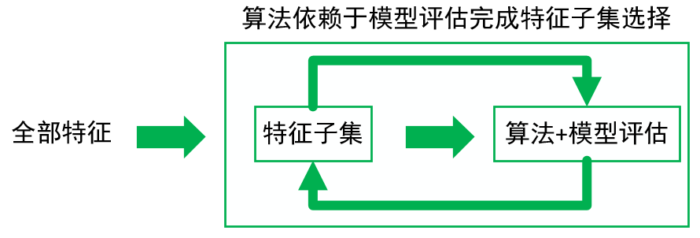feature_selection.SelectFromModel

`class sklearn.feature_selection.SelectFromModel (estimator, threshold=None, prefit=False, norm_order=1,max_features=None)`
 参数 说明 estimator 使用的模型评估器，只要是带feature_importances或者coef_属性，或带有l1 和 l2 惩罚项的模型都可以使用。 threshold 特征重要性的阈值，重要性低于这个阈值的特征都将被删除 prefit 默认False，后的模型直接传递给构造函数。如果为True，则必须直接调用fit和transform，不能使用fit_transform，并且SelectFromModel不能与cross_val_score，GridSearchCV和克隆估计器的类似实用程序一起使用。 norm_order K可输入非零整数，正无穷，负无穷，默认值为1。载频鼓起的coef_属性高于一维的情况下，用于过滤低于阈值的系数的向量的番薯的阶数。 max_features 在阈值设定下，要选择的最大特征数。要禁用阈值并仅根据max_features选择，请设置threshold = -np.inf

```from sklearn.feature_selection import SelectFromModel
from sklearn.ensemble import RandomForestClassifier as RFC
import numpy as np
import matplotlib.pyplot as plt

RFC_ = RFC(n_estimators =10,random_state=0)
print(X_embedded.shape)
#模型的维度明显被降低了
#画学习曲线来找最佳阈值

RFC_.fit(X,y).feature_importances_
threshold = np.linspace(0,(RFC_.fit(X,y).feature_importances_).max(),20)
score = []
for i in threshold:
X_embedded = SelectFromModel(RFC_,threshold=i).fit_transform(X,y)
once = cross_val_score(RFC_,X_embedded,y,cv=5).mean()
score.append(once)
plt.plot(threshold,score)
plt.show()
X_embedded = SelectFromModel(RFC_,threshold=0.00067).fit_transform(X,y)
X_embedded.shape
print(cross_val_score(RFC_,X_embedded,y,cv=5).mean())```

# 4 Wrapper包装法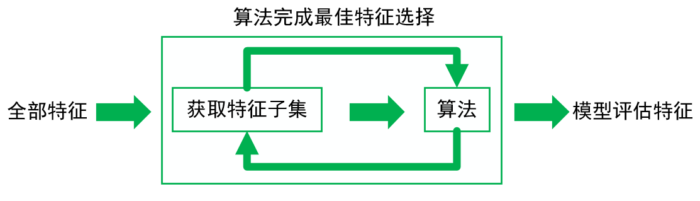feature_selection.RFE

class sklearn.feature_selection.RFE (estimator, n_features_to_select=None, step=1, verbose=0)

 参数 说明 estimator 使用的模型评估器。 n_feature_to_select 所需特征数 step 每次迭代中希望移除的特征数

RFE类中有两个比较重要的属性，.support_：返回所有的特征的是否最后被选中的布尔矩阵，以及.ranking_返回特征的按数次迭代中综合重要性的排名。类feature_selection.RFECV会在交叉验证循环中执行RFE以找到最佳数量的特征，增加参数cv，其他用法都和RFE一模一样。

```import pandas as pd
import numpy as np
import matplotlib.pyplot as plt
from sklearn.ensemble import RandomForestClassifier as RFC
from sklearn.model_selection import cross_val_score
from sklearn.feature_selection import RFE

x = data.iloc[:,1:]
y = data.iloc[:,0]
# print(x.shape)
RFC_ = RFC(n_estimators=10,random_state=0)
score = []
for i in range(1,751,50):
x_wrapper = RFE(RFC_,n_features_to_select=i,step=50).fit_transform(x,y)
once = cross_val_score(RFC_,x_wrapper,y,cv=5).mean()
score.append(once)
plt.figure(figsize=[20,5])
plt.plot(range(1,751,50),score)
plt.xticks(range(1,751,50))
plt.show()```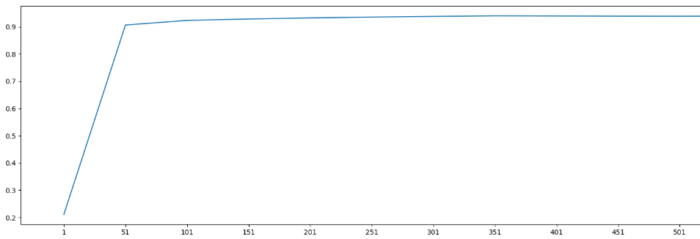# 5 总结

• 版权声明 本文源自 s1awwhy 整理 发表
• 转载请务必保留本文链接：https://www.plob.org/article/28117.html